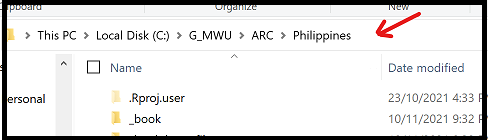# Chapter 5 IRT Item Analysis

In this session, we will learn how to

• read a file in csv format
• score a data set
• produce item distractor analysis

You will need to specify the location of the file on your computer. I find it easiest to copy the file path from Windows Explorer.Clicking on this path, Windows shows

C:\G_MWU\ARC\Philippines

To use this path, you must change the back slashes (\) to forward slashes (/). The following shows the R code for setting a working directory.

setwd("C:/G_MWU/ARC/Philippines")  # Note forward slashes

## 5.2 Exercise 1

The data file contains scored item responses of a numeracy test. The data file can be downloaded here (click on link). The test questions can be downloaded here.

rm(list=ls())

library(CTT)
library(TAM)
library(WrightMap)

#Read an item response file with scored data
setwd("C:/G_MWU/ARC/Philippines/files")  # set working directory: make sure you use forward slashes

#Run CTT item analysis
IA <- itemAnalysis(resp)
IA$alpha IA$itemReport

#Run IRT analysis
mod1 <- tam.jml(resp)
summary(mod1)
mod1$WLEreliability plot(mod1) wrightMap(mod1$WLE, mod1$xsi, item.side=itemClassic) ### 5.2.1 Answer these questions From the output of the CTT and IRT analyses, answer the following questions: • How many students are in the item response file? • How many items are in the item response file? • Which item is the easiest? Which item is the most difficult? • Which item is the least discriminating? Which item is the most discriminating? • What is the constraint set in the IRT calibration (hint: see the summary(mod1) output). What does this mean? • What is the mean item difficulties? What is the mean person abilities? • Is the test generally easy for the students, or difficult? • What is the CTT test reliability? What is the IRT test reliability? ## 5.3 Exercise 2 The item response data analysed in Exercise 1 is also provided before scoring was carried out. We will call this the raw response data file. Download this file and save it to your computer. In this data file, for each question, a range of students’ answers are recorded. For example, for Item 1, the list of answers is (2,3,4,5,6,7,8,99). As a convention, “99” is used for “all other answers.” We need to read in the raw response data file, and score the responses. In reading the data file, we will make sure that all responses are read in as characters, and not as numeric values (see read.csv options). The keys to the item responses are provided in the code block below. rm(list=ls()) library(CTT) library(TAM) library(WrightMap) key <- c("4","3 hundreds","1720","8","160mL","1100","Bir and Mif","3","15","5","2022","5","football","C","35") setwd("C:/G_MWU/ARC/Philippines/files") resp_raw <- read.csv("B1_raw_E.csv", stringsAsFactors = FALSE, colClasses = rep("character", length(key))) s <- score(resp_raw,key=key,output.scored=TRUE) #Use CTT score function to score the raw responses resp <- s$scored #The scored responses are in s$scored mod2 <- tam.jml(resp) summary(mod2) The results of the IRT estimations for Exercises 1 and 2 should be exactly the same. The only difference is that in Exercise 2 we read in a raw response file and we carried out scoring before carrying out an IRT analysis. One useful thing for reading in the raw responses (instead of the scored responses) is that we can carry out an item distractor analysis to see what kind of answers students provided, and whether high or low ability students provided a particular answer. In the TAM package, the function tam.ctt provides a distractor analysis, as shown below. ItemAnal<- tam.ctt(resp_raw,mod2$WLE)
print(ItemAnal)

For now, if you want to save the output of the item analysis, you can use the sink command.

sink("tamctt.txt")
print(ItemAnal)
sink()

## 5.4 Homework

Carry out CTT and IRT analyses for a grammar test. The test questions can be downloaded here. The data file can be downloaded here. The test is of multiple-choice format. The data file contains “A,” “B,” “C,” “D” characters, and the responses are not scored. The keys are provided below.

key <- c("D","D","D","B","B","B","A","D","C","B","A","C","D","A","B")

Carry out CTT and IRT analyses, and answer the list of questions as in 5.2.1